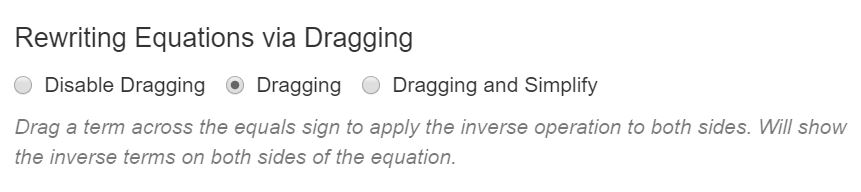# Solving Equations

Graspable Math is easy to use, I decided I would solve an equation and wanted to show all the steps. To start, go to a blank canvas and choose Insert / Math Expression, I have used the method of selecting and holding the = sign to start as you can see illustrated in the video above; I was then able to enter an operation to apply to both sides of the equation.An alternative to the above is to use the settings menu; choose Dragging to apply the inverse operation to both sides.

We can also illustrate a solution graphically by inserting a graph to open a GeoGebra window.

You can see this canvas here. Each expression has a circle at the end – simply drag that to the GeoGebra window. You will sometimes see more than one circle at the end of an expression, select to separate expressions hence showing all steps clearly.

You can insert a video on a canvas, watch it and try out the feature on the canvas. Try solving an equation on this canvas.